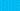Open site navigation sidebarPricing# What is the accumulated depreciation formula?

Written by

Last editedNov 20202 min read

As an asset drops in value over time, this is marked as depreciation for accounting purposes. Accumulated depreciation refers to cumulative asset depreciation up to a single point during its lifespan.

## Understanding accumulated depreciation

According to the generally accepted accounting principles (GAAP), any expenses that a business lists must be matched to any related revenue. These matching expenses and revenues must be recorded on the balance sheet during the same accounting period.

Depreciation offers a way for businesses to list a capital asset’s value as an expense. As the asset is used to generate revenue during its lifespan, any costs associated with using the asset are also recorded. This is where accumulated depreciation comes in. This figure shows the total amount that asset has depreciated, up to a fixed and single point.

A simple accumulated depreciation formula would look like this:

We’ll take a closer look at what this means below, starting with what the accumulated depreciation account is called.

## What type of account is accumulated depreciation?

Accumulated depreciation is considered a contra asset account because its balance is a credit that reduces the asset’s value. You can find the asset’s carrying value listed on the balance sheet, showing the difference between historical cost and accumulated depreciation. When the asset reaches the end of its lifespan (or the period it is of use to the company), the carrying value will match its salvage value. A simple formula for depreciation is, therefore, as follows:

During every accounting period, the depreciation expense recorded for that period is added to the accumulated depreciation balance. As we’ve touched on above, the accumulated depreciation account is called a long-term contra asset account. To record depreciation using this method, debit the depreciation expense and credit the accumulated depreciation value.

Depreciation expenses appear on the income statement during the recording period, while accumulated depreciation shows up on the balance sheet under related capitalised assets. You’ll note that the balance increases over time as depreciation expenses are added.

## How to calculate accumulated depreciation formula

There are several methods you can use when determining how to calculate the accumulated depreciation formula. Accumulated depreciation is usually calculated from month to month, so you can apply this to one of the following depreciation methods:

### Straight-line method

With the straight-line method, you use the following formula:

Adapt this to a monthly depreciation formula with these steps:

1. Subtract the asset’s salvage value from its total cost to determine what is left to be depreciated.

2. Divide this value by the number of years of the asset’s lifespan.

3. Divide this figure by 12 to learn the monthly depreciation.

### Declining balance method

Another option is the declining balance method, which weighs the asset’s depreciation more heavily upfront. This method assumes that an asset receives the most use when it’s new and applies to assets like electronic equipment or vehicles. As a result, depreciation amounts change from year to year. The double declining depreciation formula is as follows:

To convert this figure into a monthly depreciation rate, divide your result by 12.

### Sum of the years’ digits (SYD) method

The final method for calculating accumulated depreciation is the SYD or sum of the years’ digits. This formula looks like this:

To calculate the sum of the years, you need to know the projected useful life and then add these together. For example, an asset expected to last for five years would have 3 + 2 + 1 for a total of six.

No matter which method you choose, you’ll need to know some basic information to apply the accumulated depreciation formula. This includes the asset’s useful lifespan, the salvage value, and the asset’s associated costs.

## We can help

GoCardless helps you automate payment collection, cutting down on the amount of admin your team needs to deal with when chasing invoices. Find out how GoCardless can help you with ad hoc payments or recurring payments.